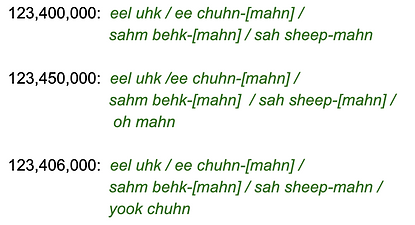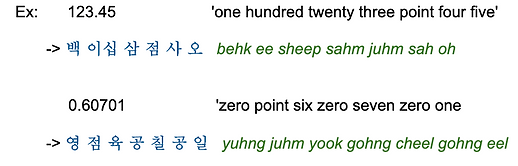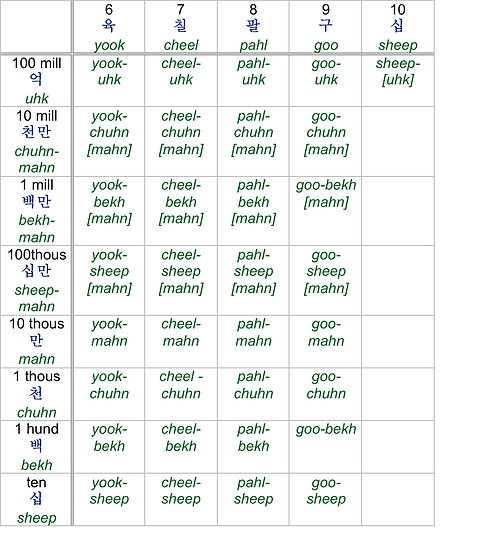###### 1-to-10-in-increments-of-1

Each expression will be repeated three times. You should repeat after each, imitating the sound and the intonation as closely as possible for better fluency. The table below is for reference only.

00:00 / 01:19###### 10-to-100-million-in-multiples-of-10

Each expression will be repeated three times. You should repeat after each, imitating the sound and the intonation as closely as possible for better fluency. The table below is for reference only.

00:00 / 01:20###### Reading  Numbers

Each expression will be repeated three times. You should repeat after each, imitating the sound and the intonation as closely as possible for better fluency. The table below is for reference only.

00:00 / 01:14
00:00 / 01:14

Let's practice reading some numbers with Chinese-origin numbers, starting with simple ones:Now, with regard to reading numbers in Korean, please note three important things explained below:

<1> When reading sheep-mahn's (hundred thousand), behk-mahn's (million), and chuhn-mahn's (ten million), the word mahn (ten thousand) is not read if there is any digit with mahn following after them. In the examples below, the mahn (=10,000) of chuhn-mahn (=10 million), behk-mahn (=1 million), and sheep-mahn (=100,000) is put in brackets to remind you that it should not be read if there is a digit with mahn following them:Thus, they are read:Here are more examples. This time, let us focus on when not to omit the word mahn. The mahn in chuhn-mahn (ten million), behk-mahn (million) and sheep-mahn (hundred thousand) is read if there is no digit with mahn following it:<2> With the same idea, uhk (=100 million) is not read in sheep-uhk (=1 billion), behk-uhk (=10 billion), and chuhn-uhk (=100 billion) when there is any digit with uhk following after them. Here are some examples. Again, the brackets are there to remind you that uhk and mahn are omitted in the situations described above and in <1>:Thus, they are read:Here are other examples. This time, uhk and mahn are read because there is no digit with uhk or mahn following them:<3> Unlike with other digits, the eel (=1) is not said with sheep (=10):###### Reading Telephone Numbers, Decimals & Scores

Chinese-origin numbers are used when reading telephone numbers, decimal numbers, and scores.<Telephone Numbers>

• Each number is usually read as a single-digit, with eh often inserted between groups of numbers, for example. between area code and the rest of the numbers.

• For zero, 공 gohng is used.<Decimal Numbers>

• The numbers on the left of the decimal point are read as they are; and the numbers on the right of the decimal point are read as single-digit numbers. Use the charts of Chinese-origin numbers on p11.

• The decimal point is read '점 juhm'. For zero, use 영 yuhng for the zero on the left of the decimal point; use 공 gohng for the zeroes on the left of the decimal point.<Scores>

• Use 대 deh between scores for 'versus' or 'to'.

• For zero, 영 yhuhng is usually used.###### Numbers In Chart
• What is in the brackets is not read if a digit containing the same term is present after it.

• The eel in the parentheses can be omitted.1 Refer to p15 for the use of 영 yuhng and 공 gohng.